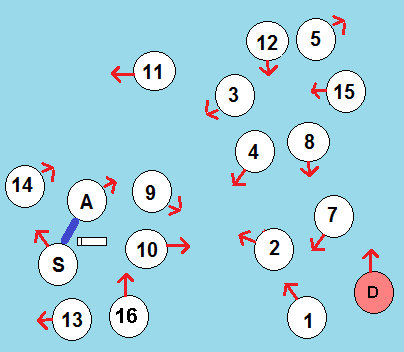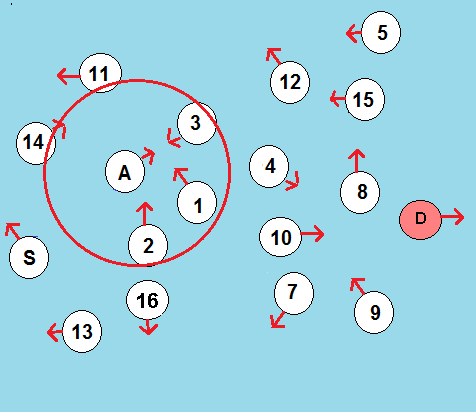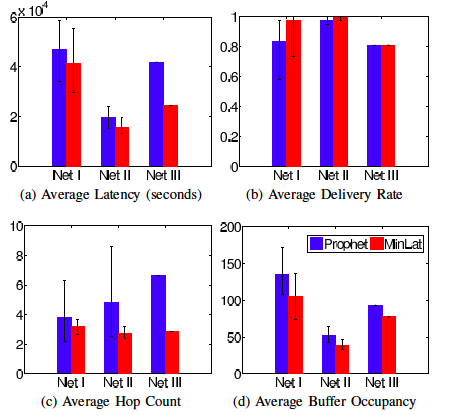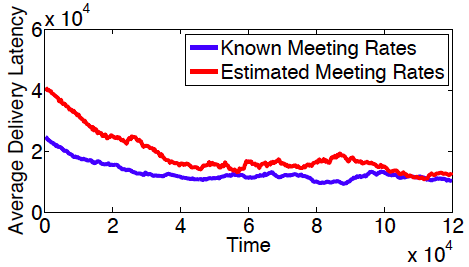## Routing in Opportunistic Networks

### Opportunistic networks are a group of communication networks in which nodes are mobile with random mobility patterns. Hence, the network topology and connectivity change dynamically and end-to-end communication paths between nodes may not exist. In these networks, it becomes important to seize opportunities to forward data to neighbouring nodes in the hope that it will eventually be propagated to the destination. Forwarding algorithms must address the trade-off between delivery performance (success rate, end-to-end delay) and resource consumption (memory and bandwidth).

The main question to answer is : When to forward a message and to whom?Look at the left figure above. The source node wants to send a message to the destination node D and it decides to forward it to node A. After some time, all the nodes have moved and the topology is as the right figure above. Now, node A should decide on whether to forward the message to some of the nodes 1, 2, or in its communication range or to hang on to it.

### Replication Based Methods:

In replication-based routing algorithms such as Epidemic and Spray and wait, a node forwards messages stored in its buffer to all or a fraction of encountered
nodes without assessing their capability to deliver them. The only parameters in these algorithms are the extent of replication and the nodes that are allowed to
make new copies. The replication approaches can achieve a higher probability of message delivery since more nodes have a copy of each message, but they
can produce significant network congestion.

### History Based Methods:

History-based routing algorithms assume that nodes' movement patterns are not completely random and that the rate of future contacts between two nodes
depend on the frequency and number of past encounters and possibly the time gaps between them. PRoPHET assigns a "delivery predictability'' metric to
each node for each destination. This metric is updated each time two nodes meet, and thus takes into account the history of meetings in the network. The
metric is intended to indicate how likely it is that this node will be able to deliver a message to that destination (perhaps via other nodes).  MaxProp and
MEED, proposed for vehicular DTNs, are other examples of this class of algorithms. 

### Social Based Methods:

Social-based algorithms incorporate the principle that mobile devices are usually carried by members of a society and therefore the contact patterns depend
on their social interactions. This suggests that social network analysis concepts and community formations can be used to better predict the node meeting
patterns and identify superior forwarding rules.  In Simbet, a node decides to forward a message to other nodes based on their betweenness centralities and
a social similarity metric. BubbleRap makes use of community affiliation and centrality to make forwarding decisions. The contact graph based routing
algorithm uses information derived from a weighted contact graph to make forwarding decisions based on the neighbourhood, community and degree centrality
of a node.




A closed network of $N$ mobile nodes is considered in which all the nodes aim to send messages to a particular destination node $d$. The set of nodes is denoted by $\mathcal{N}$. We assume that the random intermeeting times of nodes are independent and exponentially distributed with parameter $\lambda_{ij}$ for nodes $i$ and $j$. We assume that each time node $i$ meets one of its neighbors $j \in \mathcal{S}_i$, it forwards a message destined for $d$ with probability $p_{ij}$. Considering the matrix $\mathbf{P}_{N \times N}$ comprised of all pairs $i$ and $j$, we set $p_{ij}=0$ if nodes $i$ and $j$ never meet. The expected latency of a node $i \in \mathcal{N}$ to the destination $d$ is a function of the probability decision matrix $\mathbf{P}$ and we denote it by $L_{id}(\mathbf{P})$:

$L_{id}(\mathbf{P})= \frac{1+\Sigma_{k \in \mathcal{S}_i}m_{ik}\lambda_{kd}L_{kd}(i)}{\Sigma_{k \in \mathcal{S}_i}m_{ik}\lambda_{kd}}$

Our goal is to find the matrix $\mathbf{P}^*$ such that the sum of the expected latencies of all the nodes in the network to the specified destination $d$ is minimized. We propose both centralized and decentralized message forwarding algorithms that, under relatively strong assumptions about the network's behaviour, solve the following optimization problem:

$\min_{\mathbf{P}} \Sigma_{i \in \mathcal{N}} L_{id}(\mathbf{P})$

After presenting proofs of the optimality of our proposed algorithms, we confirm their improvement in system performance through numerical simulations.

 The four comparison metrics in the three test networks  using PRoPHET and MinLat algorithms are shown in this figure.The error bars in the graphs  represent the $95\%$ confidence intervals. The results indicate that  MinLat achieves a  smaller average latency than PRoPHET for the test networks. For Net I and Net II, where the assumption of exponentially distributed  intermeeting times holds, this is expected from proof. But we observe that  the average latency reduction holds also for Net III, where a power-law distribution provides a better fit to intermeeting times. The better performance of MinLat over PRoPHET in terms of average  latency is due to our primary goal in developing MinLat which  is minimizing the sum of expected latencies of all nodes to the destination node.  Also, reductions in hop count and buffer occupancy  are side effects of this minimization.The delivery rates of the algorithms  are less than 1 because the simulation is terminated before all generated  messages are successfully delivered.We conducted further simulations with a different message generation scenario to explore how the incorporation of meeting rate estimations  affects the performance of our proposed algorithm MinLat. Figure  shows the average delivery latency as a function of time. Messages are generated every $t$ seconds at randomly chosen source nodes where $t$ is uniformly distributed in $[0,1]$. As we see in the figure, the average time it takes for a message to be delivered decreases as time goes by and the forwarding rules discovered by MinLat get closer to the optimum forwarding rules. This decreasing trend is observed in both known and estimated meeting rates scenarios, but the convergence to the optimum point is slower when nodes need to estimate the meeting rates.

Ph.D. Student: Shohreh Shaghaghian

Faculty: Prof. Mark Coates

Proc. IEEE Int. Conf. on Wireless and Mob. Comput., Net. and Comm. (WiMob), Larnaca, Cyprus, Oct., 2014.